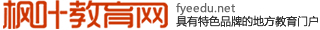《因数中间 或末尾有0的乘法》教案设计

掌握因数中间或末尾有0的计算方法，进一步认识0在乘法运算中的特.

1、探究因数末尾有0的乘法笔算

1、探究因数中间有0

1、 口算

40×72=  600×300=  30×23=

53×30=  20×700=  40×22=

40×72=  40×72=  40×72=

20×20=  40×90=  502×7=

608×5=  908×4=  400×50=

2、笔算

708×6=    790×8=  54×278=

3、这节课继续学习笔算乘法

160×30=    106×30=

1、说一说第一个算式，你是怎么计算的？

2、说一说口算的方法尝试笔算。

3、小组讨论：因数末尾有0的笔算方法和口算方法一样吗？

1）160×30=  问：写竖式时，如何处理0和非0数字的对位问题？怎样确定积的末尾0的个数？

160×30=4800

160

×  30

—————

4800

2）106×30=  自己试一试

× 30

————

（2）计算106×30时，既然中间的0与3相乘得0，那么这个过程可以不要吗？如何写这一位的积？

106×30=3180

106

×  30

——————

3180

1、书后第48页做一做

2、练习八的第3

因数中间 或末尾有0的乘法

160×30=4800                                 106×30=3180

160                                         106

×  30                                       ×  30

—————                                 —————

4800                                          3180

### 问题伴我在身边——做小课题的经验和体会

“这几年写了那么多文章，十几部著作，你给我们介绍一下经验吧！”经常有同事或博友这样问我。我的回答很简单，就是“勤奋好学，心中时时有问题，且能持之以恒去...详情